# Translations of Encyclopedia about Mathematics

## Assertion Logics

Using logics, it is possible to determine whether some mathematical deduction was correct or whether some complicated argument is true. You may be aware of logical problems, an example of which is:

Gabi is organising a social evening, to which eight guests have already promised their presence. One day before her gathering, she received a response from the following and remaining guests: Peter wants to come only if Hannelore will come; Jan promised to come (Sabina is Jan’s sister); andrea said she would come only if Peter will come; either Canny or Hannelore will come; Sabina is sick and said she could not come; Gabi’s best friend of Sack will not come if Jan and Sabina will come; and Hannelore will come only if Jan’s sister will come. Gabi will order a pizza for all those coming only if less than 10 will come. (Solution at the end of this document.)

When solving this problem, you should first organise all the information to help you formalise the solution.

To solve this problem, we use formal logic and logical connection symbols (function operators):

A A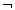A not A negation
A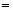B A equals B identity/equality
A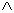B A and B conjunction
A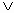B A or B disjunction or adjunction
A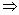B "if A, then B" implication
A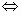B "if A, then B and if B, then A" equality/equivalence

Logics is a theory of correct thought conclusions. Logical conclusions are composed of premises (postulates) and conclusions (results).

For example:

1. premise: All people are mortal
2. premise: Socrates is a person
Conclusion: Socrates is mortal

If we would like to write down this argument using formal logical symbols:

P1 AB
P2 A
K B

AB "is a person who is mortal"
A " Socrates is a person"
B " Socrates is mortal"

For logics, only statements which may be true or false and which have a clear truth value are interesting for us. For our example above, this means that the conclusion is true only if all the premises are true. To make things more understandable, we can work with so-called Tables of Truth Values. Instead of writing "truth", we write p or the number 1; and instead of "false" we write the letter l or the number 0.

Negation

A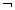A
p
l
l
p

If A is true, then NOT A is not true and vice versa. If A means that Jan has rusty coloured hair, then NOT A means that Jan does not have rusty coloured hair.

Conjunction: the joining statement and

A
B
A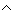B
p
p
p
p
l
l
l
p
l
l
l
l

If A means "Jan has rusty coloured hair" and B means "Jan is a sporty person", then unification of these two means that Jan has rusty coloured hair and is a sporty person if both A and B are true, which would make the statement A B true. However, if one of the premises were not true, then the conjunction A B would also not be true.

Disjunction: or

The joining statement or can have two different meanings:

1) Disjunctive: Jan has rusty or black coloured hair (either/or)

A
B
A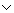B
p
p
l
p
l
p
l
p
p
l
l
l

2) Nondisjunctive: Jan is small or sports oriented (can be both)

A
B
AB
p
p
p
p
l
p
l
p
p
l
l
l

Implication: if A then B (from A ensues B)

A
B
A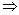B
p
p
p
p
l
l
l
p
p
l
l
p

"A implicates B" can always be expressed as "if A, then B" because "if-then" statements express a correlation between the factual content of two statements.

For example: "When it will rain, the soil will be wet." (Which can be written as: Rainsthe ground will be wet.)

An implication can have no correlation between its first statement A and its second statement B.

Example: "3 time 3 is 6.""Earth is a planet"

As with a consequential statement using an if-then connection, the following implication is also not correct: "If 3 times 3 equals 6, then the Earth is a planet." In any case, the implication is not correct and is false if A is true and B is false. Pure logical implication is always true if A is false regardless of whether B is true or false.

Equivalence: A equals B

A
B
A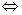B
p
p
p
p
l
l
l
p
l
l
l
p

The statement AB is always true if A and B have the same truth values. A equals B can also be expressed as follows: A implies B and B implies A. Here, the same difference between implication and the standard if-then statement applies: if A then B and if B then A. We can also say: "A occurs only if B occurs." With pure logical equivalence, no factual relation needs to exist between the statements A and B.

Example: Each is a planetOcean currents affect our climate.

If both these statements are true, then their equivalence is also true. If both statements are false, then their equivalence is, once again, also true.

Example: The earth is squareThe climate is controlled by the actions of the tree-frog.

Using these logical connections, we can formalise proofs. However, it is also necessary to make sure that the expressions are correct so that logical relations can be correctly determined between the individual statements. As in algebra and in the case of sets, logical relations can be reformulated, abbreviated and re-calculated. Logical-mathematical considerations are subject to an entire system of rules with which logical problems can be solved systematically. By formal means, complicated functions can also be solved.

Logics is therefore a useful tool which enables us to test the accuracy of deduced conclusions, procedures which have made the likes of Sherlock Holmes and Hercules Poiret famous for their excellent logic.

Another form of logic is predicative logic. With this type of logic, quantors are used: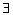x means "at least one x exists" and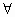x means "all x" relating to the predicates or characteristics refer to relations between certain objects.

Following is how some statements can be written formally:

Some cows have a mottled pelt.

There exists at least one x for which it applies that: is mottled.x F(x)

All warm-blooded creatures have a heart.

For all x it applies that: have a heart.x F(x)

Marie loves Franta.

Someone loves someone else.

There exists at least one x and at least one y for which it applies that: x loves y.xy G(x, y)

Using predicative logics, logical statements can be evaluated and relations can be reformulated. However, the contents of these statements can be worked with to a much greater degree than with assertion logics.

Invitation to a Social Evening

8 guests will come for certain. Important: the implication corresponds to the consequential statements with the joining if-then statement.

Peter = p
Hannelore = h
Jan = j
Sabina= s
andrea = a
Conny = c
Zack = z
Number of guests = n
Pizza for all = i
Sabina is sick and cancelled her participation.s

s = false

1

Sabina is Jan’s sister.

Important for 2
Hannelore wants to come only in the event that Jan’s sister comes.
Hannelore will not come.

sh

s = false
refer to 1
therefore: h = false

2

Either Conny or Hannelore will come.

Conny will come.
ch

h = false
refer to 2
therefore: c = true

3

Peter wants to come only in the event that Hannelore will come.

Peter will not come.
ph

h = false
refer to 2
therefore: p = false

4

andrea will come only if Peter will come.

andrea will not come.
pa

p = false
refer to 4
therefore: a = false

5

Jan promised today that he will come.

j
j = true

6

Gabi’s best friend Zach will not come if both Jan and Sabina come.

Zach will come.
(js)z

s = false
refer to 1
(js) = false
therefore: z = true

7

Gabi will order a pizza for everyone if less than 10 guests will come.

Gabi will not order a pizza.
n < 10i

n = 11 > 10
n < 10 = false
therefore: i = false

8

Peter = p
Hannelore = h
Jan = j
Sabine= s
andrea = a

Conny = c
Zack = z
8 old + 3 new guests = 11 guests total
Number of guests = n = 11 Pizza for everyone = i = false

Translating Dutch Hungarian Translations Hungarian Dutch Translating Swedish Czech Translations Czech Swedish Translating RussianEnter your search terms Submit search form